# How to use Regex in Pandas

There are several pandas methods which accept the regex in pandas to find the pattern in a String within a Series or Dataframe object. These methods works on the same line as Pythons re module. Its really helpful if you want to find the names starting with a particular character or search for a pattern within a dataframe column or extract the dates from the text.

Here are the pandas functions that accepts regular expression:

Methods Description
count() Count occurrences of pattern in each string of the Series/Index
replace() Replace the search string or pattern with the given value
contains() Test if pattern or regex is contained within a string of a Series or Index. Calls re.search() and returns a boolean
extract() Extract capture groups in the regex pat as columns in a DataFrame and returns the captured groups
findall() Find all occurrences of pattern or regular expression in the Series/Index. Equivalent to applying re.findall() on all elements
match() Determine if each string matches a regular expression. Calls re.match() and returns a boolean
split() Equivalent to str.split() and Accepts String or regular expression to split on
rsplit() Equivalent to str.rsplit() and Splits the string in the Series/Index from the end

## Create Dataframe

First create a dataframe if you want to follow the below examples and understand how regex works with these pandas function

``````import pandas as pd
``````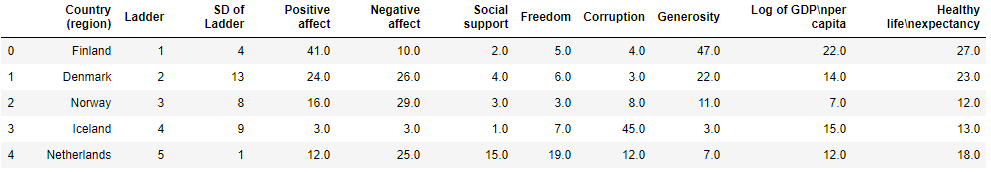## Pandas extract

Extract the first 5 characters of each country using ^(start of the String) and {5} (for 5 characters) and create a new column first_five_letter

``````import numpy as np
df['first_five_Letter']=df['Country (region)'].str.extract(r'(^w{5})')
``````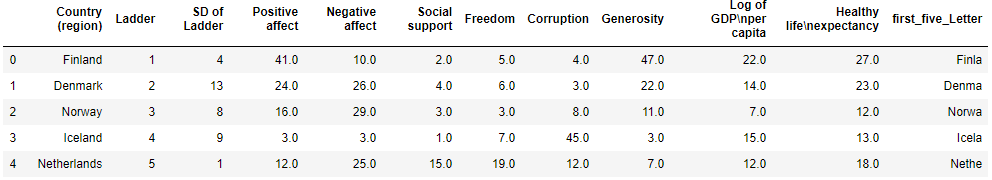## Pandas Count

First we are counting the countries starting with character ‘F’. It returns two elements but not france because the character ‘f’ here is in lower case. you can add both Upper and Lower case by using [Ff]

``````S=pd.Series(['Finland','Colombia','Florida','Japan','Puerto Rico','Russia','france'])
S[S.str.count(r'(^F.*)')==1]
``````

Output:

0 Finland 2 Florida dtype: object

OR

We can use sum() function to find the total elements matching the pattern.

``````# Total items starting with F
S.str.count(r'(^F.*)').sum()
``````

Output: 2

In our Original dataframe we are finding all the Country that starts with Character ‘P’ and ‘p’ (both lower and upper case). Basically we are filtering all the rows which return count > 0.

``````df[df['Country (region)'].str.count('^[pP].*')>0]
``````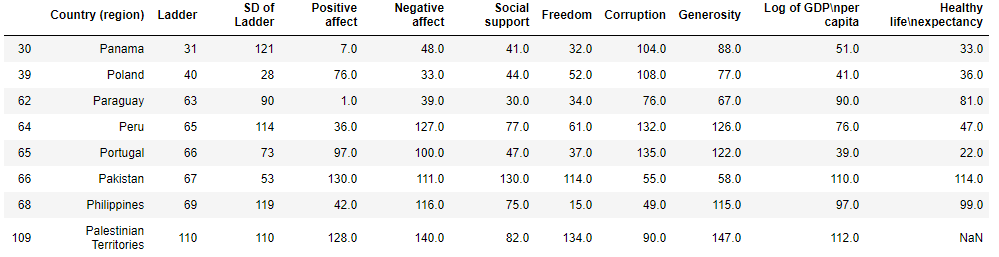## Pandas Match

match () function is equivalent to python’s re.match() and returns a boolean value. We are finding all the countries in pandas series starting with character ‘P’ (Upper case) .

``````# Get countries starting with letter P
S=pd.Series(['Finland','Colombia','Florida','Japan','Puerto Rico','Russia','france'])
S[S.str.match(r'(^P.*)')==True]
``````

Output: 4 Puerto Rico

Running the same match() method and filtering by Boolean value True we get all the Countries starting with ‘P’ in the original dataframe

``````df[df['Country (region)'].str.match('^P.*')== True]
``````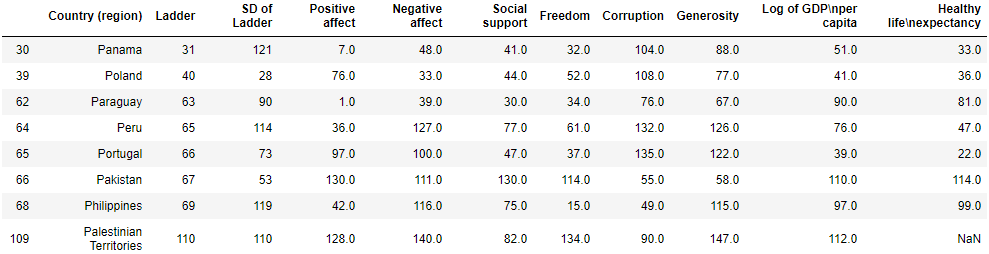## Pandas Replace

Replaces all the occurence of matched pattern in the string. We want to remove the dash(-) followed by number in the below pandas series object. The regex checks for a dash(-) followed by a numeric digit (represented by d) and replace that with an empty string and the inplace parameter set as True will update the existing series. The output is list of countres without the dash and number.

``````# Remove the dash(-) followed by number from all countries in the Series
S=pd.Series(['Finland-1','Colombia-2','Florida-3','Japan-4','Puerto Rico-5','Russia-6','france-7'])
S.replace('(-d)','',regex=True, inplace = True)
``````

Output:

0 Finland 1 Colombia 2 Florida 3 Japan 4 Puerto Rico 5 Russia 6 france

## Pandas Findall

It calls re.findall() and find all occurence of matching patterns. We are creating a new list of countries which starts with character ‘F’ and ‘f’ from the Series. The list comprehension checks for all the returned value > 0 and creates a list matching the patterns.

``````S=pd.Series(['Finland','Colombia','Florida','Japan','Puerto Rico','Russia','france'])
[itm for itm in S.str.findall('^[Ff].*') if len(itm)>0]
``````
``````**Output:** ['Finland', 'Florida', 'france']
``````

## Pandas Contains

It uses re.search() and returns a boolean value. In the below regex we are looking for all the countries starting with character ‘F’ (using start with metacharacter ^) in the pandas series object. The result shows True for all countries start with character ‘F’ and False which doesn’t.

``````S=pd.Series(['Finland','Colombia','Florida','Japan','Puerto Rico','Russia','france'])
S.str.contains('^F.*')
``````

0 True 1 False 2 True 3 False 4 False 5 False 6 False

In our original dataframe we will filter all the countries starting with character ‘I’ . We just need to filter all the True values that is returned by contains() function.

``````df[df['Country (region)'].str.contains('^I.*')==True]
``````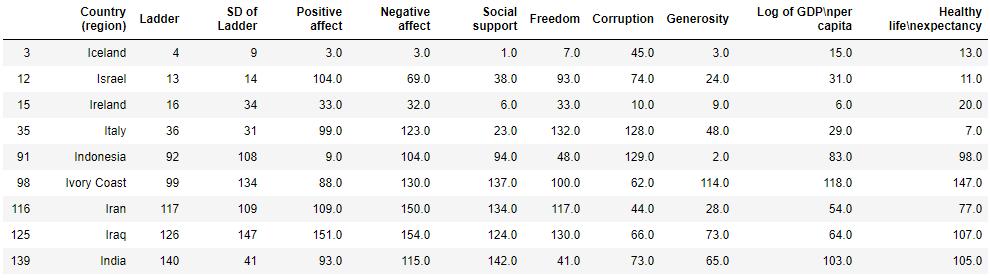## Pandas Split

This is equivalent to str.split() and accepts regex, if no regex passed then the default is \s (for whitespace). Here we are splitting the text on white space and expands set as True splits that into 3 different columns

You can also specify the param n to Limit number of splits in output

``````s = pd.Series(["StatueofLiberty built-on 28-Oct-1886"])
s.str.split(r"s", n=-1,expand=True)
``````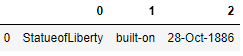## Pandas rsplit

it is equivalent to str.rsplit() and the only difference with split() function is that it splits the string from end.

## Conclusion

We have seen how regexp can be used effectively with some the Pandas functions and can help to extract, match the patterns in the Series or a Dataframe. Especially when you are working with the Text data then Regex is a powerful tool for data extraction, Cleaning and validation.

Tags:

Categories:

Updated: